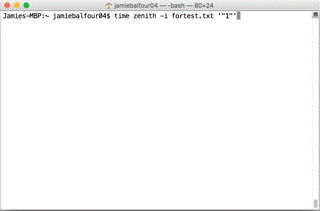# Improving the speed and efficiency of a program

Over the last few years, I've done a lot of work on building my own programming language and platform known collectively as ZPE.

Perhaps the most important lesson I have learned from this is how to make a program more efficient. I focus a lot on shifting things from the interpreter/runtime side to the compile-time side in ZPE, which has been a major focus of the latest version. However, there are some things that I cannot do very easily.

I recently started thinking about making one of my programs more efficient and how this would work in ZPE. Let's take a look at some code:

YASS
$l = range(1, 5000) //For x is less than the length of the list (i.e. 5000), increment by 1 for ($x = 0, $x < list_get_length($l), 1)
print($x) end for  Notice how we check the size of the list at the top of the for? This means each iteration will need to call that function to find the size of the array. If a variable had been defined before the for loop and contained the length of the list, one could simply reference the variable, which in turn would be much faster than constantly asking the system to find the length of a 5,000 element array. Here is a sample of this in action. YASS $l = range(1, 5000)
$len = list_get_length($l)
//For x is less than the length of the list (i.e. 5000), increment by 1
for ($x = 0,$x < $len, 1) print($x)
end for


Times were measured using the Unix time command and were as follows:

For the first test:

real 0m1.821s

user 0m2.862s

sys 0m0.363s

And for the second test:

real 0m0.437s

user 0m1.051s

sys 0m0.099s

This is the first tip I have for you. This tip will also work in other languages such as JavaScript or Java or whatever.## In 2020

In 2020 when I was looking through my blog, I came across this post and thought I'd test it out again for a bit of fun. Interestingly, running both for loops is considerably faster than even the faster for loop example provided here. Compiler optimisations, runtime improvements and much more have made this much faster in ZPE 1.8.5. The version shown above is running on ZPE 1.4.2E, which is still available to download and compare.

efficient
efficiency
programming
speed
fasterfficient
efficiency
programming
speed
faster